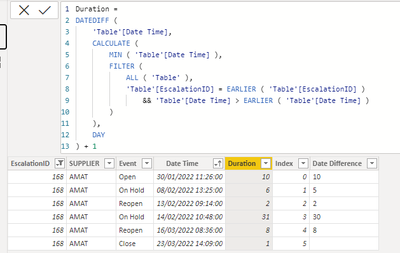cancel
Showing results for
Did you mean:Helper III

## Event duration

Hi all,

I have a question, i have this table, and im trying to know how long spesific escalation was in each event type. For example- escalation 168 opened-->moved to on hold-->reopened---> moved to on hold again---> reoepened again and then closed.

i want to know for how long it was on hold and open?1 ACCEPTED SOLUTIONSuper User

Hi @gkakun ,

You are correct, I have made the contrary logic how long does it take to get from the previous status.

Replace the calculations by:

``````Duration =
DATEDIFF (
'Table'[Date Time],
CALCULATE (
MIN ( 'Table'[Date Time] ),
FILTER (
ALL ( 'Table' ),
'Table'[EscalationID] = EARLIER ( 'Table'[EscalationID] )
&& 'Table'[Date Time] > EARLIER ( 'Table'[Date Time] )
)
),
DAY
) + 1``````

``try if  #"Added Index"[EscalationID]{[Index] + 1} = [EscalationID] then  Duration.Days( ( #"Added Index"[Date Time]{[Index] + 1}) - [Date Time]) + 1 else null otherwise null``Regards

Miguel Félix

Proud to be a Super User!

Check out my blog: Power BI em Português4 REPLIES 4Helper III

Thanks a lot! its working.Super User

Hi @gkakun ,

``````Duration =
DATEDIFF (
CALCULATE (
MAX ( 'Table'[Date Time] ),
FILTER (
ALL ( 'Table' ),
'Table'[EscalationID] = EARLIER ( 'Table'[EscalationID] )
&& 'Table'[Date Time] < EARLIER ( 'Table'[Date Time] )
)
),
'Table'[Date Time],
DAY
)``````

This is in days but you can the use it in hours months, and so on.

Now just use the status to get the sum of that value:You can also do this on M Query:

• Sort the table by EscalationID and then by Data time
• Add the following calculated column:
``try if  #"Added Index"[EscalationID]{[Index] - 1} = [EscalationID] then  Duration.Days( ([Date Time] - #"Added Index"[Date Time]{[Index] - 1})) else null otherwise null``

Result below:Has you can see below both of the columns have similar results:The variations has to do with the time conversion on DAX and Power Query.

Regards

Miguel Félix

Proud to be a Super User!

Check out my blog: Power BI em PortuguêsHelper III

Hi Friend,

Thanks a lot for your help.

The logic is not working well. For example, escalation number 168 was on hold from Feb 8 until Feb 13 (on hold, and then reopened, 5 days), and then was o hold from Feb 14 until March 16, and then reopened, and closedf on March 23 (32 days). Overall 37 days and not 10Super User

Hi @gkakun ,

You are correct, I have made the contrary logic how long does it take to get from the previous status.

Replace the calculations by:

``````Duration =
DATEDIFF (
'Table'[Date Time],
CALCULATE (
MIN ( 'Table'[Date Time] ),
FILTER (
ALL ( 'Table' ),
'Table'[EscalationID] = EARLIER ( 'Table'[EscalationID] )
&& 'Table'[Date Time] > EARLIER ( 'Table'[Date Time] )
)
),
DAY
) + 1``````

``try if  #"Added Index"[EscalationID]{[Index] + 1} = [EscalationID] then  Duration.Days( ( #"Added Index"[Date Time]{[Index] + 1}) - [Date Time]) + 1 else null otherwise null``Regards

Miguel Félix

Proud to be a Super User!

Check out my blog: Power BI em Português